Analysis WebNotes
arrow_back arrow_forward

# Class Contents

## Arithmetic with Convergent Sequences

### Overview

The main result of this section shows that the limits of sums, products, and quotients of convergent sequences carry over as the sums, products and quotients of the limits. This lets us do algebra with convergent sequences. The main result of this section is Theorem 3.9. However, all the work that we need to do to prove this theorem is built up in the lemmas and propositions that precede it. When you get to 3.9, you'll find it doesn't even have a proof!

An important application of Theorem 3.9 is to prove that nth roots of positive real numbers exist. That's in Theorem 3.10.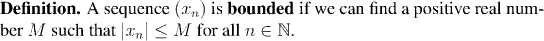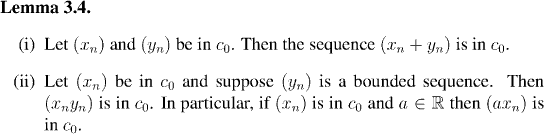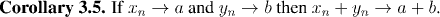Remark:

• If (xn) converges to a, and we take (yn) to be a constant sequence of -a's then this result supersedes Lemma 3.2.
• Another way to phrase this result is: if (xn) and (yn) are convergent sequences then
`lim (xn + yn) = lim xn  +  lim yn`
Before we can prove the next arithmetic result, we need a quick result on convergent sequences; if a sequence converges, then it is bounded. This isn't surprising, since if a sequence is getting closer and closer to a fixed number, then it can't very well be getting uncontrollably large or small at the same time. Still, it needs a little proof, and to make the proof go smoothly, we need a useful alternative criterion for telling when a sequence is bounded:

Factoid: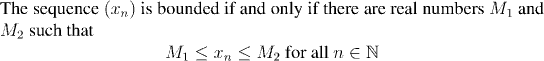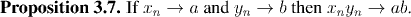The last arithmetic result we need to prove is that if xn converges to a and yn converges to b, then xn/yn converges to a/b (at least, as long as b is non-zero). Since xn/yn=x_n (1/yn), it is enough (by Proposition 3.7) to show that 1/yn converges to 1/b.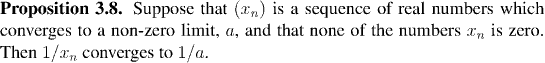Finally, we summarize the results of this section in a theorem: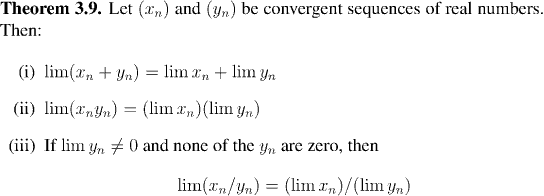Example:Remember how much work it was back in Chapter 2 to prove that the set s of positive rational numbers which squared to less than 2 was non-empty, bounded above and that its supremum, a satisfied a2=2?

We needed to do that work to prove that there really was a real number which was the square root of 2. And since we knew that no rational number could square to 2, we also got from that result that irrational real numbers did exist. We can now generalize that result considerably, and at the same time avoid all the difficult technical estimates that made the earlier result so hard.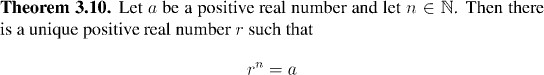Remark:Let x be a positive real number. We know how to define xn when n is a natural number (it's just the product of n copies of x). We know how to define x(-n) when n is a natural number (it's just the reciprocal of xn).

Now we also know how to define x(1/n), where n is a natural number. One of the big themes in this course will be the development of this pattern, first to the point when we define xy, where y is a real number, then for a complex number. A lot of analysis will have to flow under the bridge before we completely understand how that works....